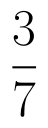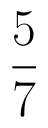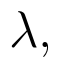Select Page

# MCQ in English Probability Answers for CBSE Maths 12 Science

MCQ in English Probability Answers for CBSE Maths 12 Science to enable students to get Answers in a narrative video format for the specific question.

Expert Teacher provides MCQ Probability Answers for CBSE Maths 12 Science through Video Answers in English language. This video solution will be useful for students to understand how to write an answer in exam in order to score more marks. This teacher uses a narrative style for a question from Probability not only to explain the proper method of answering question, but deriving right answer too.

Please find the question below and view the Answer in a narrative video format.

Question:

## Similar Questions from CBSE, 12th Science, Maths, Probability

Question 1 : One card is drawn from a pack of 52 cards. Find the probability of getting a jack of hearts:

Question 2 : A box contains 50 bolts and 50 nuts. Half of the bolts and nuts are rusted. If two items are drawn with replacement, what is the probability that either both are rusted or both are bolts.    (View Answer Video)

Question 3 : The probabilities of two students A and B coming to the school in time areandrespectively. Assuming that the events ' A coming in time ' and
' B coming in time ' are independent. Find the probability of only one. Write of them come in time atleast one advantage of coming to school in time.   (View Answer Video)

Question 4 : How many times must a man toss a fair coin, so that the probability of having at least one head is more than 80%.  (View Answer Video)

Question 5 :  In a group of 400 people, 160 are smokers and non-vegetarian, 100 are smokers and vegetarian and the remaining are non-smokers and vegetarian. The probabilities of getting a special chest disease are 35%, 20% and 10% respectively. A person is chosen from the group at random and is found to be suffering from the disease. What is the probability that the selected person is a smoker and non-vegetarian? What value is reflected in this question?     (View Answer Video)

### Vector Algebra

Question 1 : Write the position vector of the point which divides the join of points with position vectorsandin the ratio 2:1.  (View Answer Video)

Question 2 : Find the unit vector in the direction ofifand(View Answer Video)

Question 3 : Find the vector quantities from the following:
(i) Time period          (ii) Distance          (iii) Force
(iv) Velocity               (v) Work done

Question 4 : Findif the vectorsandare coplanar.   (View Answer Video)

Question 5 : Find the angle between two vectors a and b with magnitudesand 2 respectively, having(View Answer Video)

### Linear Programming

Question 1 : The objective function is maximum or minimum, which lies on the boundary of the feasible region. (View Answer Video)

### Inverse Trigonometric Functions

Question 1 : Ifthen find the value of x. (View Answer Video)

Question 2 :is equal to :
Question 3 : What is principal value of(View Answer Video)
Question 4 :is equal to : (View Answer Video)(View Answer Video)